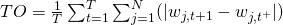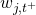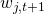# Portfolio turnover

What does turnover rate mean in mutual funds? Portfolio turnover is an important concept in finance that is related to the active management of an investment portfolio. The portfolio turnover is sometimes also called the asset turnover ratio, investment turnover or the equity turnover. It gives investors some insight into how often securities in the portfolio that’s being analysed, are bought and sold. As such, the turnover rate can help investors to get an idea on the number of transactions that they will need to execute to apply a certain investment strategy. That’s why this ratio it an important element that’s often reported when one backtests trading strategies. Many mutual funds also report their turnover. In that case, the mutual fund’s turnover rate gives investors an idea of how actively the mutual fund manager is managing the portfolio. Now, let’s go over how we can calculate an investment’s turnover ratio.

## Turnover definition

There are several ways in which we can define investment turnover. Most often, investment turnover is expressed as an annual figure. In that case, we talk about annual turnover. However, the turnover of a portfolio can also be reported on a monthly, or even as a daily rate. The fund turnover ratio formula looks as followswhereis the portfolio weight of security j before rebalancing at t+1 andthe portfolio weight after rebalancing. T equals the number of periods, and N equals the total number of securities.

The above portfolio turnover formula produces a percentage which can be interpreted as the average fraction of the portfolio value that has been been bought or sold over the period T. In this case, TO is the portfolio turnover ratio. The more active the investment strategy, the higher the turnover will be. A buy and hold strategy will have a turnover of zero.

## Interpretation

As a general rule, a low turnover is preferable to a high turnover ratio. This is because trading involves transaction costs and taxes. Passive investing approaches tend to have low turnover rates, whereas active investment approaches can have rates of over a 100%. In that case, on average, the portfolio manager performs transactions whose notional value over the period exceeds the value of the portfolio.

The turnover ratio can also tell us something about the average holding period of the securities in the portfolio. For example, a turnover ratio of 50% implies that the average holding period of a security is two years. Similarly, a mutual fund that reports turnover rate of 200% only holds stocks for half a year, on average.

## Summary

The portfolio turnover rate indicates how much the portfolio changes over a certain period. It indicates how actively managed the underlying portfolio is. Portfolios with a higher turnover ratio however also bear more transaction costs. This diminishes the returns to investors and should thus kept in mind.